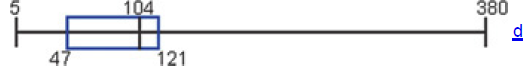×
Get Full Access to Elementary Statistics - 12 Edition - Chapter 3.4 - Problem 3bsc
Get Full Access to Elementary Statistics - 12 Edition - Chapter 3.4 - Problem 3bsc

×

# Boxplots Shown below is a STATDISK-generated boxplot ofISBN: 9780321836960 18

## Solution for problem 3BSC Chapter 3.4

Elementary Statistics | 12th Edition

• Textbook Solutions
• 2901 Step-by-step solutions solved by professors and subject experts
• Get 24/7 help from StudySoup virtual teaching assistantsElementary Statistics | 12th Edition

4 5 1 339 Reviews
24
0
Problem 3BSC

Boxplots Shown below is a STATDISKgenerated boxplot of the amounts of money (in millions of dollars) that movies grossed (based on data from the Motion Picture Association of America). What do the displayed values of 5, 47, 104, 121, and 380 tell us?Step-by-Step Solution:
Step 1 of 3

Problem 3BSC

Step1 of 3:

We have Shown below is a STATDISK-generated boxplot of the amounts of money (in millions of dollars) that movies grossed (based on data from the Motion Picture Association of America).Step2 of 3:

We need to find what do the displayed values of  5, 47, 104, 121, and 380 tell us?

Step3 of 3:

From the above box plot we can say that the lowest amount is $5 million, the first quartile Q1 is$47 million, the second quartile Q2 (or median) is $104 million, the third quartile Q3 is$121 million, and the highest gross amount is \$380 million.

Step 2 of 3

Step 3 of 3

##### ISBN: 9780321836960

Elementary Statistics was written by and is associated to the ISBN: 9780321836960. Since the solution to 3BSC from 3.4 chapter was answered, more than 361 students have viewed the full step-by-step answer. This textbook survival guide was created for the textbook: Elementary Statistics, edition: 12. The answer to “?Boxplots Shown below is a STATDISKgenerated boxplot of the amounts of money (in millions of dollars) that movies grossed (based on data from the Motion Picture Association of America). What do the displayed values of 5, 47, 104, 121, and 380 tell us?” is broken down into a number of easy to follow steps, and 43 words. The full step-by-step solution to problem: 3BSC from chapter: 3.4 was answered by , our top Statistics solution expert on 03/15/17, 10:30PM. This full solution covers the following key subjects: America, amounts, association, based, below. This expansive textbook survival guide covers 121 chapters, and 3629 solutions.

Unlock Textbook Solution